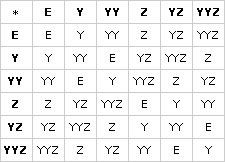# Algebraic Structure Homework

## Session 9, Homework

### Problems

Problem H1

In this game, starting with a string of Ys and Zs, the object is to simplify the string by following strict rules. The rules are:

 • YYY can be erased. • ZZ can be erased. • The commutative law holds: YZ = ZY. • E is the empty string (a string with no Ys or Zs).Example 1: Step 1: YZZYYZYZYYZ (first erase ZZ) Step 2: Y YYZYZYYZ (erase YYY) Step 3: ZYZYYZ (commute YZ) Step 4: ZZYYYZ (erase ZZ and YYY) Step 5: Z (can’t be simplified)Example 2: Step 1: ZYYYZ (erase YYY) Step 2: ZZ (erase ZZ) Step 3: E (empty string is left)Simplify the following strings:

 a. YZYZZYYZ b. YYYYZZYZY c. YZYZYZYZYZYZYZYZZZYZYZYYZY

Problem H2

Including the empty string E, there are six essentially different strings that cannot be simplified. They are called the elements of the YZ group.

Find all the elements of the YZ group.

Problem H3

The symbol “*” represents the operation “put together and simplify.” For example:

 • YZ * YZ = YY • Y * E = Y

Compute:

 a. E * YZ b. YZ * YY c. Z * YZ

Problem H4

Find the missing term.

 a. YZ * __ = E b. Z * __ = YZ c. YY * __ = Z

Problem H5

For the YZ group, * works a little bit like multiplication. Another way to write the first two rules is Y3 = E and Z2 = E. Explain.

Problem H6

The only powers of Y are Y, Y2, and E. Explain.

Problem H7

Find all the powers of each element of the YZ group.

Problem H8

Simplify:

 a. Y1,000 b. (YZ) 1,001

Problem H9

Make a * table.

Problem H10

What element of the group works like the number 1 for multiplication?

Problem H11

What is the reciprocal of each element?

### Solutions

Problem H1

a:

 Step 1: YZYZZYYZ Step 2: YZYYYZ (erase ZZ) Step 3: YZZ (erase YYY) Step 4: Y (erase ZZ)

b:

 Step 1: YYYYZZYZY Step 2: YZZYZY (erase YYY) Step 3: YYZY (erase ZZ) Step 4: YYYZ (commute last ZY) Step 5: Z (erase YYY)

c:

 Step 1: YZYZYZYZYZYZYZYZZZYZYZYYZY (better think more systematically) Step 2: YYYYYYYYYYYYYZZZZZZZZZZZZZ (commute all Ys first, Zs last) Step 3: YZ (erase 12 Ys by threes, 12 Zs by twos)

Problem H2

The elements of the YZ group are E, Y, YY, Z, YZ, and YYZ.

Problem H3

Y3 means the same as Y * Y * Y, which is YYY, which is the same as E. The same is true of Z2, which is identical to Z * Z.

Problem H4

Y3 means the same as Y * Y * Y, which is YYY, which is the same as E. The same is true of Z2, which is identical to Z * Z.

Problem H5

Y3 means the same as Y * Y * Y, which is YYY, which is the same as E. The same is true of Z2, which is identical to Z * Z.

Problem H6

Since Y3 is identical to E, Y4 will be identical to Y, Y5 = YY, Y6 = E, etc.

Problem H7

Powers of each element:

 • E: E only • Y: Y1 = Y, Y2 = YY, Y3 = E • YY: YY1 = YY, YY2 = Y, Y3 = E • Z: Z1 = Z, Z2 = E • YZ: YZ1 = YZ, YZ2 = YY, YZ3 = Z, YZ4 = Y, YZ5 = YYZ, YZ6 = E • YYZ: YYZ1 = YYZ, YYZ2 = Y, YYZ3 = Z, YYZ4 = YY, YYZ5 = YZ, YYZ6 = E

Problem H8

 a. Y1,000 = Y1, since 1,000 = 1 (mod 3), and the powers of Y repeat every three powers. b. (YZ)1,001 = (YZ)5, since 1,001 = 5 (mod 6), and the powers of YZ repeat every six powers. According to the list of powers of YZ, (YZ)5 = YYZ. Another way to do this is to imagine a line of 1,001 Ys and 1,001 Zs, and decide what would be left after all the cancellation.

Problem H9

Here is the completed table:Note that every element appears exactly once in each row, and once in each column.

Problem H10

The element E works this way, since E * A = A for any element A in the table, just like 1 * N = N for any number N.

Problem H11

The reciprocals can be found by finding E within the row and column of each element. Here are the reciprocals, in pairs:

 • E and E • Y and YY • Z and Z • YZ and YYZ